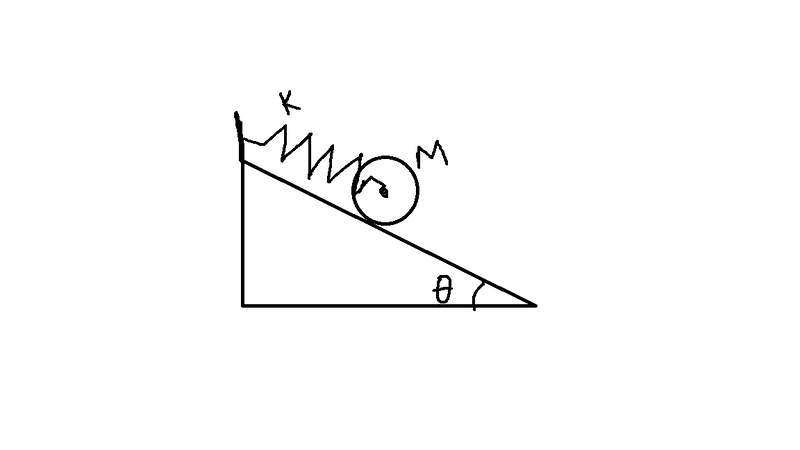# Rotational Mechanics question with spring

## Homework StatementA uniform cylinder of mass ##M## and radius ##R## is released from rest on a rough inclined surface of inclined surface of inclination ##\theta## with the horizontal as shown in the figure. As the cylinder rolls down the inclined surface, what is the maximum elongation in the spring of stiffness ##k##?

2. The attempt at the solution
Here is what I have thought about the problem:

We choose the origin to coincide with the equilibrium position of the spring, the x-axis along the incline and the y-axis perpendicular to it.

Let ##a## be the linear acceleration at an arbitrary instant of time. Also let ##\alpha## be the angular acceleration of the block at the same. Since there is no slipping, we have ##a=R\alpha##.

The equation for tangential motion is, therefore $$Mg\sin\theta - kx-f=Ma$$

For angular motion, $$fR=I\alpha$$

Where,
##x =## displacement from the origin
##g =## acceleration due to gravity
##f =## force of friction
##I =## moment of inertia of the cylinder through its central axis

Substituting the value of ##f## from the second equation into the first, we obtain $$mg\sin\theta - kx- \frac{I\alpha}{R} = Ma$$

But at the extreme position, linear as well as tangential acceleration is zero. Substituting, ##a=\alpha=0## in the above we get $$x=\frac{Mg\sin\theta}{k}$$

But this is the elongation from the origin, and the spring was initially at ##-x##. Therefore maximum elongation is given by$$x_{max} = \frac{2Mg\sin\theta}{k}$$

Am I correct in my reasoning? The answer is given to be this but I suspect I might have missed something in the middle as I am new to rotational mechanics. Any help would be appreciated. Thanks a lot.

#### Attachments

Orodruin
Staff Emeritus
Homework Helper
Gold Member
2021 Award
It is a bit unclear to me what you mean by the spring's equilibrium position. You could mean one of two things:
• Where the system is at equilibrium.
• Where the spring is unstretched.
Your argumentation in the end seems to suggest the first, but your argumentation for the location of the zero-acceleration point seems to suggest the latter.

That being said, you could also just do an energy argument, which will avoid any rotational involvement altogether.

It is a bit unclear to me what you mean by the spring's equilibrium position. You could mean one of two things:
• Where the system is at equilibrium.
• Where the spring is unstretched.
Your argumentation in the end seems to suggest the first, but your argumentation for the location of the zero-acceleration point seems to suggest the latter.

That being said, you could also just do an energy argument, which will avoid any rotational involvement altogether.
I'm sorry for being unclear. I meant the position where the spring is unstretched.

Orodruin
Staff Emeritus
Homework Helper
Gold Member
2021 Award
Then that is where the contraption was released so
But this is the elongation from the origin, and the spring was initially at −x.
is not true. The spring was initially at ##x = 0##. However, the point you have computed is not that where the system changes direction, it is where it has zero acceleration.

Then that is where the contraption was released so

is not true. The spring was initially at ##x = 0##. However, the point you have computed is not that where the system changes direction, it is where it has zero acceleration.
I see that flaw. Thanks a lot for pointing it out. I found a better and neater solution.

The decrease in gravitional potential energy is equal to the increase in spring potential energy and hence we have $$mgx\sin\theta = \frac{1}{2}kx^2$$
Rearranging yields the answer. I hope this is correct :)